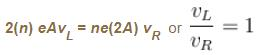Courses

# Current Electricity NAT Level - 2

## 10 Questions MCQ Test Topic wise Tests for IIT JAM Physics | Current Electricity NAT Level - 2

Description
This mock test of Current Electricity NAT Level - 2 for Physics helps you for every Physics entrance exam. This contains 10 Multiple Choice Questions for Physics Current Electricity NAT Level - 2 (mcq) to study with solutions a complete question bank. The solved questions answers in this Current Electricity NAT Level - 2 quiz give you a good mix of easy questions and tough questions. Physics students definitely take this Current Electricity NAT Level - 2 exercise for a better result in the exam. You can find other Current Electricity NAT Level - 2 extra questions, long questions & short questions for Physics on EduRev as well by searching above.
*Answer can only contain numeric values
QUESTION: 1

### Figure shows a cell in which unit positive charge experience a constant non electric force of 10N  and a constant electric force of 8N  in directions shown in the figure. Find the emf of the cell, difference across the cell (in volt)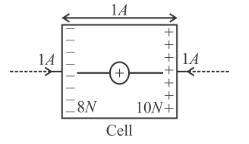Solution:

V = Potential difference across the cell = Electric field × width of the cell
= 8 × 0.1 = 0.8 volt
ε = emf of the cell = 10 × 0.1 = 1.0 volt

*Answer can only contain numeric values
QUESTION: 2

### In the circuit diagram shown if the current through the 1Ω resistor is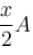then x is :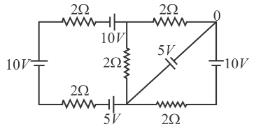Solution: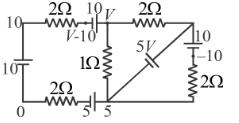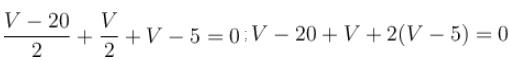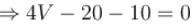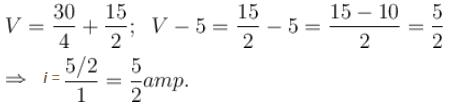*Answer can only contain numeric values
QUESTION: 3

### Consider the potentiometer circuit arranged as in figure. The potentiometer wire AB  is 300cm long. If the jockey touches the wire at a distance of 275cm from A, then (3E/Nr) current flow through galvanometer. Find value of  N.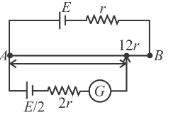Solution:

When Jockey is not connected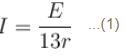Resistance per unit length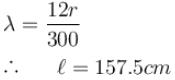Let potential at C  is zero
Then apply Kirchoff’s Ist law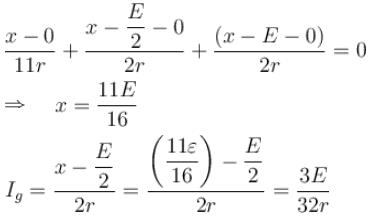*Answer can only contain numeric values
QUESTION: 4

The circuit shown in the figure contains three resistors R1 =100Ω, R2 = 50Ω, and R3 = 50Ω and cells of emf’s E1 = 2V and E2. The ammeter indicates a current of 50mA. Determine the current (mA) in the resistor R2 :

Solution: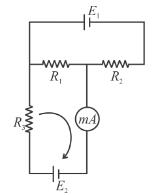Applying KVL in loop ABCD
E1 = (I + 0.05) R1 + IR2
I = –20mA
Current through R1 = 30mA towards right
Current through R2 = 20mA towards left
Applying KVL in loop BGFE
E2 = (I + 0.05) 100 + (0.05) 20 = 4 volts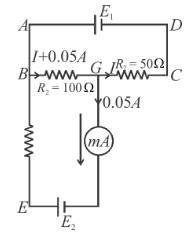*Answer can only contain numeric values
QUESTION: 5

The efficiency of a cell when connected to a resistance R  is 60%. What will be its efficiency (in %) if the external resistance is increased to six times.

Solution: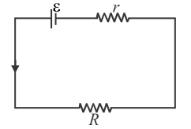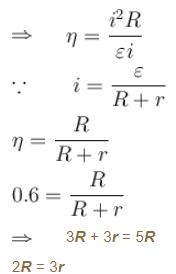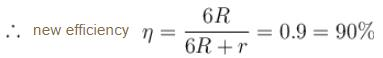*Answer can only contain numeric values
QUESTION: 6

Find current in the branch CD of the circuit (in ampere).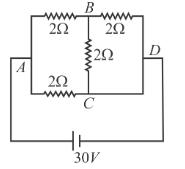Solution: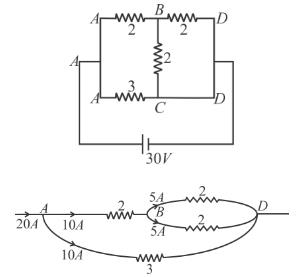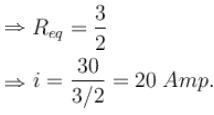From figure current through BD  branch = 5 Amp.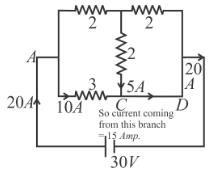*Answer can only contain numeric values
QUESTION: 7

Two circular rings of identical radii and resistance of 36Ω each are placed in such a way that they cross each other’s centre C1 and C2 as shown in figure. Conducting joints are made at intersection points  and  of the rings. An ideal cell of emf 20 volts is connected across AB. The power delivered (in watt) by cell is :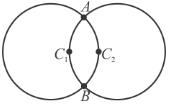Solution:

From the figure.
AC1 = AC2 = C1C2 = radius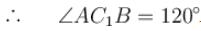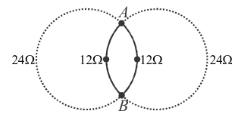Hence the resistance of four section are
Hence equivalent resistance R  across AB  is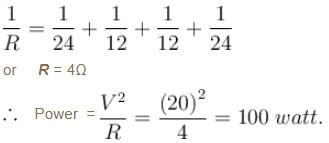*Answer can only contain numeric values
QUESTION: 8

In the circuit shown all five resistors have the same value 200 ohms and each cell has an emf 3 volts, if the open circuit voltage is x/10 volt then x is :

Solution: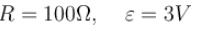In open circuit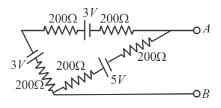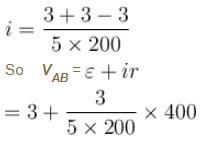VAB = 4.2V

*Answer can only contain numeric values
QUESTION: 9

All batteries are having emf  10 volt and internal resistance negligible. All resistors are in ohms. Calculate the current in the right most 2Ω resistor.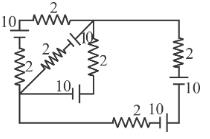Solution: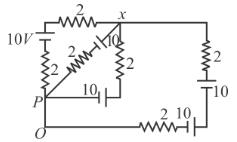The simplified circuit is.
We have to find  I.
Let potential of point ‘P’ be ‘0’. Potential at other points are shown in the figure apply kirchoff’s current law at point  x.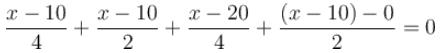⇒  x – 10 + 2x – 20 + x – 20 + 2x – 20 = 0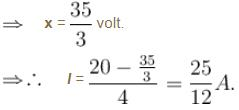*Answer can only contain numeric values
QUESTION: 10

Two cylinderical rods of uniform cross-section area A and 2A, having free electrons per unit volume 2n and n respectively are joined in series. A current I flows through them in steady state. Then the ratio of drift velocity of free electron in left rod to drift velocity of electron in the right rod is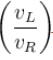is :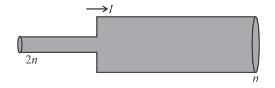Solution:

Since current I = neAvd  through both rods is same